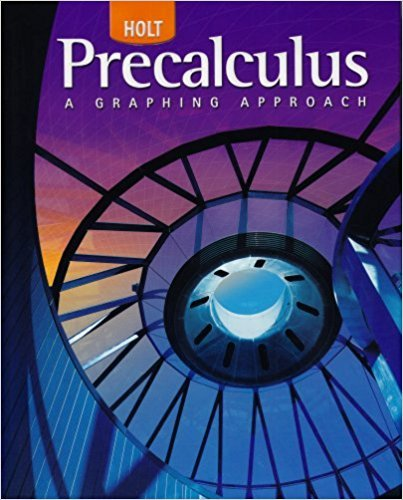×
×

# Solutions for Chapter 10.6 A: Excursion: The Dot Product## Full solutions for Precalculus | 1st Edition

ISBN: 9780030416477Solutions for Chapter 10.6 A: Excursion: The Dot Product

Solutions for Chapter 10.6 A
4 5 0 387 Reviews
22
3
##### ISBN: 9780030416477

This textbook survival guide was created for the textbook: Precalculus, edition: 1. Chapter 10.6 A: Excursion: The Dot Product includes 20 full step-by-step solutions. This expansive textbook survival guide covers the following chapters and their solutions. Precalculus was written by and is associated to the ISBN: 9780030416477. Since 20 problems in chapter 10.6 A: Excursion: The Dot Product have been answered, more than 26048 students have viewed full step-by-step solutions from this chapter.

Key Calculus Terms and definitions covered in this textbook
• artesian coordinate system

An association between the points in a plane and ordered pairs of real numbers; or an association between the points in three-dimensional space and ordered triples of real numbers

• Binomial

A polynomial with exactly two terms

• Correlation coefficient

A measure of the strength of the linear relationship between two variables, pp. 146, 162.

• Deductive reasoning

The process of utilizing general information to prove a specific hypothesis

• Exponent

See nth power of a.

• Finite sequence

A function whose domain is the first n positive integers for some fixed integer n.

• Function

A relation that associates each value in the domain with exactly one value in the range.

• Geometric sequence

A sequence {an}in which an = an-1.r for every positive integer n ? 2. The nonzero number r is called the common ratio.

• Increasing on an interval

A function ƒ is increasing on an interval I if, for any two points in I, a positive change in x results in a positive change in.

• Instantaneous velocity

The instantaneous rate of change of a position function with respect to time, p. 737.

• Inverse relation (of the relation R)

A relation that consists of all ordered pairs b, a for which a, b belongs to R.

• Inverse variation

See Power function.

• Leibniz notation

The notation dy/dx for the derivative of ƒ.

• Magnitude of a vector

The magnitude of <a, b> is 2a2 + b2. The magnitude of <a, b, c> is 2a2 + b2 + c2

• Odd-even identity

For a basic trigonometric function f, an identity relating f(x) to f(-x).

• One-to-one rule of exponents

x = y if and only if bx = by.

The measure of an angle in radians, or, for a central angle, the ratio of the length of the intercepted arc tothe radius of the circle.

The distance from a point on a circle (or a sphere) to the center of the circle (or the sphere).

• Sphere

A set of points in Cartesian space equally distant from a fixed point called the center.

• Statistic

A number that measures a quantitative variable for a sample from a population.

×